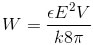﻿ All the formulas related to Electrostatics

# List of formulas related to Electrostatics

#### Coulomb's Law

q: size of charges(c), ε: relative permittivity (in vaccum ε=1), r: distance between the charges(m)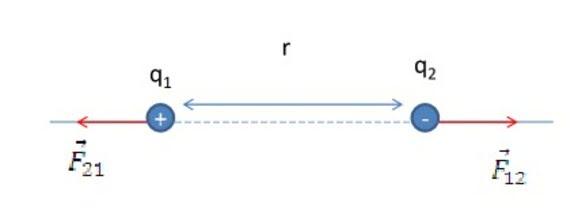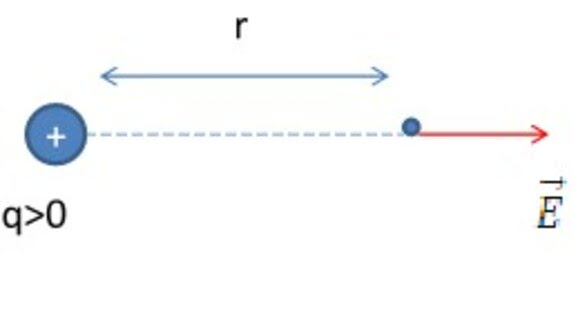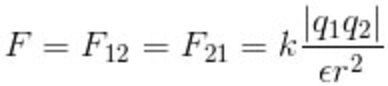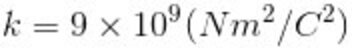#### Electric Fields

E: electric field strength (N/C)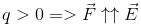#### The Principle Of Superposition For electric Fields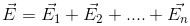In case of 2 electric fields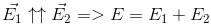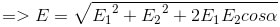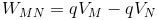#### Work Of Electric Force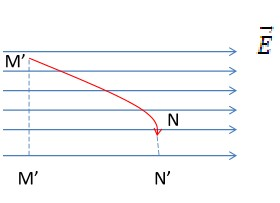#### Electric Potential Difference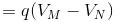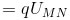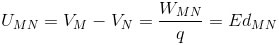#### Relation Between E And U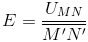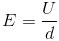#### Capacitance

C: capacitance (F), Q: total electric charge(c), U: electric potential (V)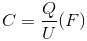#### Capacitor

A: are of each electrode plate (m2), d: distance between the electrodes (m), ε: relative permittivity (F/m)#### Capacitances In Series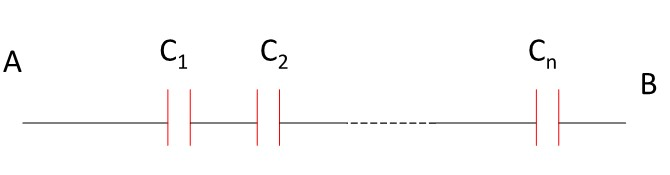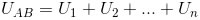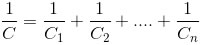#### Capacitances In Parallel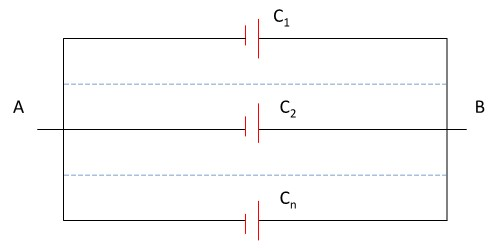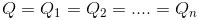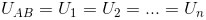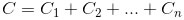#### Electric Field Energy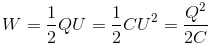#### Energy Of A Capacitor

V: A.d: volume between two electrode plates